Warning: A non-numeric value encountered in /home/triang47/public_html/Layout.php on line 233

# Acute angles

Warning: Creating default object from empty value in /home/triang47/public_html/Example_Generic.php(41) : eval()'d code on line 1

Warning: Creating default object from empty value in /home/triang47/public_html/Example_Generic.php(41) : eval()'d code on line 1
Sizes of acute angles in the right-angled triangle are in the ratio 1: 3. What is size of the larger of them?
Warning: A non-numeric value encountered in /home/triang47/public_html/Example_Generic.php on line 1037

Result

x =  67.5 °

#### Solution:

x+y=90
3 y = x

x+y = 90
x-3y = 0

x = 1352 = 67.5
y = 452 = 22.5

Calculated by our linear equations calculator.

Leave us a comment of example and its solution (i.e. if it is still somewhat unclear...):Be the first to comment!## Next similar examples:

1. Isosceles triangleWhat are the angles of an isosceles triangle ABC if its base is long a=5 m and has an arm b=4 m.
2. Triangle P2Can triangle have two right angles?
3. Complementary angles 2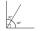Two complementary angles are (x+4) and (2x - 7) find the value of x
4. Smallest internal angle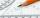Calculate what size has the smallest internal angle of the triangle if values of angles α:β:γ = 3:4:8
5. Angles ratioThe internal angles of a triangle are in ratio 1:4:5 What kind of triangle is it? (solve internal angles and write down and discuss)
6. AnglesIn the triangle ABC, the ratio of angles is: a:b = 4: 5. The angle c is 36°. How big are the angles a, b?
7. Obtuse angle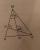The line OH is the height of the triangle DOM, line MN is the bisector of angle DMO. obtuse angle between the lines MN and OH is four times larger than the angle DMN. What size is the angle DMO? (see attached image)
8. 30-60-90The longer leg of a 30°-60°-90° triangle measures 5. What is the length of the shorter leg?
9. Plane IIA plane flew 50 km on a bearing 63degrees20 and the flew on a bearing 153degrees20 for 140km. Find the distance between the starting point and the ending point
10. A kite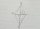ABCD is a kite. Angle OBC = 20° and angle OCD = 35°. O is the intersection of diagonals. Find angle ABC, angle ADC and angle BAD.
11. Height 2Calculate the height of the equilateral triangle with side 38.
12. Type of triangle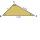How do I find the triangle type if the angle ratio is 2:3:7 ?The shadow of the tree is 16 meters long. Shadow of two meters high tourist sign beside standing is 3.2 meters long. What height has tree (in meters)?The median of the triangle LMN is away from vertex N 84 cm. Calculate the length of the median, which start at N.It is true that the middle traverse bisects the triangle?Find how many donuts each student will receive if you share 126 donuts in a ratio of 1:5:8The cylinder-shaped container contains 80 liters of milk. Milk level is 45 cm. How much milk will in the container, if level raise to height 72 cm?